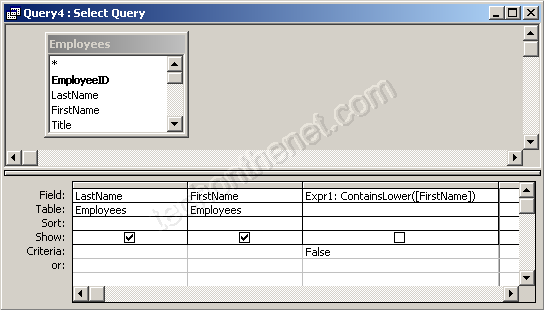## 8/16/2012

### View only records with uppercase letters for a certain field in Access

Question: In Access 2003/XP/2000/97, if I wanted to view only the records with Uppercase letters for a certain field, how would I do it?
For example, the query would ignore "Bob", but show "JOHN".
Answer: Access was built to be case-INSENSITIVE, but we can always use the ASC function to determine upper vs lower case.

To query on case-sensitivity, we've created a function called ContainsLower. You will need to paste the following function into a Module in your Access database:
Function ContainsLower(pValue) As Boolean
Dim LLength As Integer
Dim LPos As Integer
'Check for null value
If IsNull(pValue) = False Then
'Find length of string
LLength = Len(pValue)
LPos = 1
While LPos <= LLength
'Check if value is between 'a' to 'z'
If Asc(Mid(pValue, LPos, 1)) >= 97 And Asc(Mid(pValue, LPos, 1)) <= 122 Then
ContainsLower = True
Exit Function
End If
LPos = LPos + 1
Wend
End If
ContainsLower = False
End Function

This function will return FALSE if the value passed into the function contains all uppercase. It will return TRUE if at least one of the characters is NOT uppercase.
Next, you'll need to use this function in your query.In the example above, we only want records where the FirstName field contains all uppercase. So we've typed "ContainsLower([FirstName])" in the field. Access assigns the field name of "Expr1" - you can always overwrite this.
Then we've typed FALSE in the criteria (we only want uppercase values returned).
Finally, we've unchecked the Show checkbox because we don't need to see what the ContainsLower function returns - we are only using this function in our "where clause".